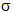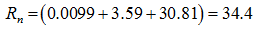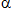you are here:/ News & Insights / Engineering Advantage Blog / How Do I Calculate Fatigue in a Random Vibration Environment? - Part 2

# How Do I Calculate Fatigue in a Random Vibration Environment? - Part 2December 11, 2015 By: Eric Stamper

In Part 1 of this post, I discussed a method for calculating fatigue damage and how to apply it to a Random Vibration load history.  In this post, I'll walk through a sample calculation using the method explained in Part 1. I'll also discuss other methods, which can produce better correlation to results for a random response.

The data needed to use the method from Part 1 is as follows:

1) The RMS (1) stress. This is a direct output from the Finite Element Analysis and used to calculate the 2and 3values (i.e. 1=5ksi, 2=10ksi, and 3=15ksi)

2) The S-n curve for the material. Some considerations one might make when using this data is the following:

a. The curve should be in the form of stress amplitude (not maximum stress).

b. It can be assumed that for a zero-mean stationary random process that the induced stress will be composed of both positive and negative peaks which corresponds to a fully reversible stress cycle. The S-n curve should then be adjusted for an R ratio of R= -1.

c. The S-n curve should account for any knockdowns, e.g. adjust the curve to the -3level (see this blog post for more information on how to do this), surface finish, etc.

3) The number of applied cycles that the component is exposed to over time (n). This can be calculated by employing the following post-processing technique.

a. We first need to determine what the statistical average frequency (expected frequency in cycles per time) is for the location on the model that's of interest. Since the FEA fatigue evaluation is performed in the frequency domain, we can calculate this quantity by dividing the velocity solution by the displacement solution (for a given stress quantity and convert the result to frequency in cycles per unit time, e.g. f=ω/2π). This represents the number of positive zero crossings of the process per unit time, and for a narrow band stationary process, each positive zero crossing implies one cycle of vibration.

b. Once the statistical average frequency is calculated, and for our example let's say it's 500Hz, we can multiply it by the time duration of the input signal to compute the statistical average number of cycles. If this component is exposed to a 2 hr test on a shaker table, the number of cycles for our location of interest would be 500Hz * 7,200sec [2hrs] = 3.6e6 cycles.

We now have all the information that's needed to calculate the fatigue damage, Rn, based on the Steinberg 3-band method. Using our previous 1stress, we can look up on the S-n curve, Figure 3, the number of cycles to failure and find that N1 = 2.5e8 cycles, and repeat for N2 and N3.

## fatigue vib3.pngFilling out the Steinberg 3-band method from Figure 2 in Part 1, we determine that Rn = 34.4.This component would therefore fail to meet the requirement of Rn being less than 1. Note that most of the damage comes from the 3 stress level, which is typical of this approach for metals. This Rn value also corresponds to a mean time to failure of 3.5 minutes (the test duration divided by the value in parentheses above). It's also worth noting that if the mean S-n data was used, Rn would equal 0.01 with a mean time to failure of 200 hrs (however there would be a 50/50 chance that the material used in this component would have these properties).

Steinberg’s method is useful for illustrating the basic concept of fatigue analysis for random processes, but it has a couple of drawbacks which hinder its accuracy. One is that the stress levels are lumped into 3 baskets of 1, 2, 3, when in reality they are distributed in amplitude. The other is the assumption that each positive zero crossing implies a cycle of vibration, which is fine for narrow band response, but is too conservative for a wide band response.

Many other frequency-domain methods are available, which produce much better correlation with rainflow-counting results for wide-band random response. Some of these include Wirsching-Light, the0:75 method, Gao-Moan, Dirlik, Zhao-Baker, Tovo-Benasciutti and Petrucci-Zuccarello. Reference 2 presents a good summary of these methods and compares the approaches to experimental methods using the rainflow-counting method with the Palmgren-Miner summation rule. The conclusion in this work was that the Dirlik, Tovo-Benasciutti and Zhao-Baker methods should be considered as the preferred methods for fatigue analysis in the frequency domain. However, this paper is certainly not the last word on random vibration damage, and other methods have been successfully implemented in various industries.

What methods have you used in your industry to calculate fatigue damage? I'm curious to hear about them!